NEET  >  Test: Bohr's Model for Hydrogen Atom

# Test: Bohr's Model for Hydrogen Atom

Test Description

## 20 Questions MCQ Test Chemistry Class 11 | Test: Bohr's Model for Hydrogen Atom

Test: Bohr's Model for Hydrogen Atom for NEET 2023 is part of Chemistry Class 11 preparation. The Test: Bohr's Model for Hydrogen Atom questions and answers have been prepared according to the NEET exam syllabus.The Test: Bohr's Model for Hydrogen Atom MCQs are made for NEET 2023 Exam. Find important definitions, questions, notes, meanings, examples, exercises, MCQs and online tests for Test: Bohr's Model for Hydrogen Atom below.
Solutions of Test: Bohr's Model for Hydrogen Atom questions in English are available as part of our Chemistry Class 11 for NEET & Test: Bohr's Model for Hydrogen Atom solutions in Hindi for Chemistry Class 11 course. Download more important topics, notes, lectures and mock test series for NEET Exam by signing up for free. Attempt Test: Bohr's Model for Hydrogen Atom | 20 questions in 20 minutes | Mock test for NEET preparation | Free important questions MCQ to study Chemistry Class 11 for NEET Exam | Download free PDF with solutions
 1 Crore+ students have signed up on EduRev. Have you?
Test: Bohr's Model for Hydrogen Atom - Question 1

### The electron in Bohr’s model of hydrogen atom is pictured as revolving around the nucleus in order for it to

Detailed Solution for Test: Bohr's Model for Hydrogen Atom - Question 1

Revolving electrons possess energy.

Test: Bohr's Model for Hydrogen Atom - Question 2

### Given below are the spectral lines for an atom of hydrogen. Mark the lines which are not correctly matched with the value of n1 and n2?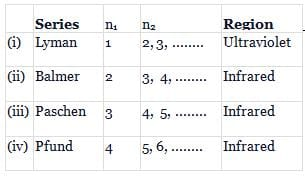Detailed Solution for Test: Bohr's Model for Hydrogen Atom - Question 2

(ii) Balmer, n1 = 2 ; n2 = 3, 4 ; region - visible
(iv) Pfund, n1 = 5 ; n2 = 6, 7; region - infrared

Test: Bohr's Model for Hydrogen Atom - Question 3

### What is the maximum number of emission lines when the excited electron of a hydrogen atom in n = 6 drops to ground state?

Detailed Solution for Test: Bohr's Model for Hydrogen Atom - Question 3

Maximum number of emission lines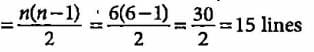Test: Bohr's Model for Hydrogen Atom - Question 4

What is the maximum number of emission lines obtained when the excited electron of a H atom in n = 5 drops to the ground state?

Detailed Solution for Test: Bohr's Model for Hydrogen Atom - Question 4

Number of lines produced when electron from nth shell drops,to ground state is given by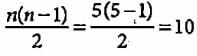Test: Bohr's Model for Hydrogen Atom - Question 5

What is the colour corresponding to the wavelength of light emitted when the electron in a hydrogen atom undergoes transition from n = 4 to n = 2?

Detailed Solution for Test: Bohr's Model for Hydrogen Atom - Question 5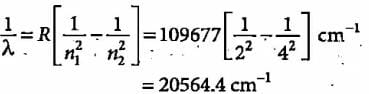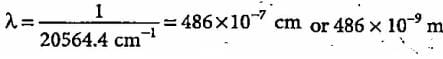Colour corresponding to this wavelength is blue.

Test: Bohr's Model for Hydrogen Atom - Question 6

_____series of lines are the only lines in hydrogen spectrum which appear in the visible region.

Detailed Solution for Test: Bohr's Model for Hydrogen Atom - Question 6

In Balmer series, the lines appear in visible region.

Test: Bohr's Model for Hydrogen Atom - Question 7

An electron in excited hydrogen atom falls from fifth energy level to second energy level. In which of the following regions, the spectrum line will be observed and is part of which series of the atomic, spectrum?

Detailed Solution for Test: Bohr's Model for Hydrogen Atom - Question 7

For Balmer series, n1 = 2, n2 = 3, 4,  5,....
The spectral lines are seen in visible region.

Test: Bohr's Model for Hydrogen Atom - Question 8

The third line of the Balmer series in the emission spectrum of the hydrogen atom is due to the transition from the

Detailed Solution for Test: Bohr's Model for Hydrogen Atom - Question 8

Rydberg’s formula for calculation of wavenumbers of hues in hydrogen spectrum is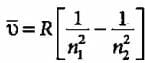For Balmer series, n1 = 2 and n2 = 3, 4, 5, so third line of the Balmer series is due to transition from fifth Bohr orbit to second Bohr orbit

Test: Bohr's Model for Hydrogen Atom - Question 9

The angular momentum of an electron in a given stationary state can be expressed as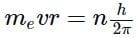Based on this expression an electron can move only in those orbits for which its angular momentum is?

Detailed Solution for Test: Bohr's Model for Hydrogen Atom - Question 9

An electron can move only in those orbit for which angular momentum is a multiple of h/2π. n = 1,2,3,...

Test: Bohr's Model for Hydrogen Atom - Question 10

According to Bohr’s theory, the angular momentum of an electron in 5th orbit is

Detailed Solution for Test: Bohr's Model for Hydrogen Atom - Question 10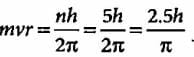Test: Bohr's Model for Hydrogen Atom - Question 11

The radius of the stationary state which is also called Bohr radius is given by the expression rn = n2a0 where the value of a0 is

Detailed Solution for Test: Bohr's Model for Hydrogen Atom - Question 11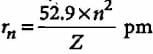Test: Bohr's Model for Hydrogen Atom - Question 12

If the radius of first Bohr orbit is x pm, then the radius of the third orbit would be

Detailed Solution for Test: Bohr's Model for Hydrogen Atom - Question 12

r = x × n2 or (9 × x) pm

Test: Bohr's Model for Hydrogen Atom - Question 13

What does the negative electronic energy (negative sign for all values of energy) for hydrogen atom means?

Detailed Solution for Test: Bohr's Model for Hydrogen Atom - Question 13

The negative sign means that the energy of an electron iii an atom is lower than the energy of a free electron at rest. When electron-is free from the influence of nucleus, the energy is taken as zero. When the electron is attracted by the nucleus, the energy is emitted and lowered.

Test: Bohr's Model for Hydrogen Atom - Question 14

If the ionisation energy of hydrogen atom is 13.6 eV, the energy required to excite it from ground state to the next higher state is approximately

Detailed Solution for Test: Bohr's Model for Hydrogen Atom - Question 14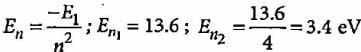Test: Bohr's Model for Hydrogen Atom - Question 15

Bohr’s theory can also be applied to the ions like

Detailed Solution for Test: Bohr's Model for Hydrogen Atom - Question 15

Bohr’s atomic theory is applicable to hydrogen and monoatomic ions like He+, Li2+, Be3+, etc.

Test: Bohr's Model for Hydrogen Atom - Question 16

According to Bohr’s theory, the electronic energy of H-atom in Bohr’s orbit is given by

Detailed Solution for Test: Bohr's Model for Hydrogen Atom - Question 16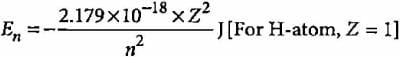Test: Bohr's Model for Hydrogen Atom - Question 17

Which of the following is not correctly matched?

Detailed Solution for Test: Bohr's Model for Hydrogen Atom - Question 17

Energy of 1 mole of photons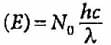where N0 is Avogadro’s number, h = Planck’s constant, c is speed of light and λ is wavelength.

Test: Bohr's Model for Hydrogen Atom - Question 18

What is the trend of energy of Bohr’s orbits?

Detailed Solution for Test: Bohr's Model for Hydrogen Atom - Question 18

Energy of the orbit increases as we move away from the nucleus or as the value of n increases.

Test: Bohr's Model for Hydrogen Atom - Question 19

The radius of hydrogen atom in ground state is 0.53 Å. What will be the radius of 3Li2+ in the ground state?

Detailed Solution for Test: Bohr's Model for Hydrogen Atom - Question 19

Radius of orbit is given by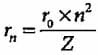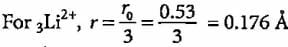Test: Bohr's Model for Hydrogen Atom - Question 20

What is the velocity of electron present in first Bohr orbit of hydrogen atom?

Detailed Solution for Test: Bohr's Model for Hydrogen Atom - Question 20

From Bohr’s theory,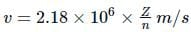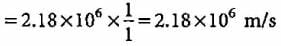## Chemistry Class 11

204 videos|331 docs|229 tests
 Use Code STAYHOME200 and get INR 200 additional OFF Use Coupon Code
Information about Test: Bohr's Model for Hydrogen Atom Page
In this test you can find the Exam questions for Test: Bohr's Model for Hydrogen Atom solved & explained in the simplest way possible. Besides giving Questions and answers for Test: Bohr's Model for Hydrogen Atom, EduRev gives you an ample number of Online tests for practice

## Chemistry Class 11

204 videos|331 docs|229 tests

### How to Prepare for NEET

Read our guide to prepare for NEET which is created by Toppers & the best Teachers